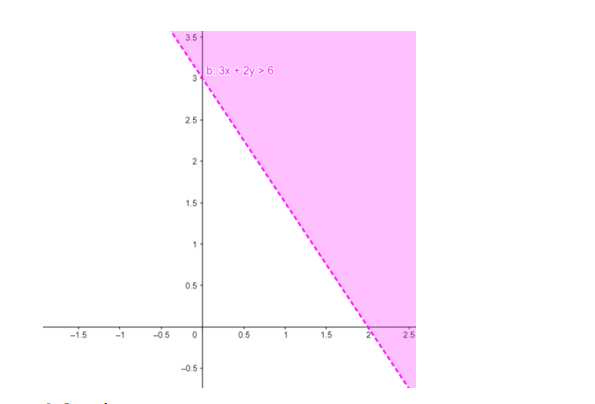# Solve the given inequality

Question:

Solve the given inequality $3 x+2 y>6$ graphically in two - dimensional plane.

Solution:

The graphical representation of $3 x+2 y>6$ is given by blue dotted line in the figure below.

This lines divides $x-y$ plane into two parts.

Select a point (not on the line), which lies on one of the two parts, to determine whether the point satisfies the given inequality or not.

We select the point as $(0,0)$

It is observed that $0+0>6$ or $0>6$ which is false.

Therefore, the solution for the given inequality excluding the points on the line.

This can be represented as follows,